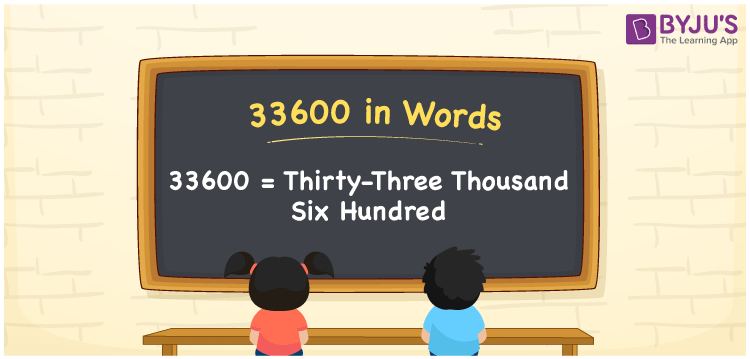# 33600 in Words

Thirty-three thousand six hundred is the word for the number 33600. The number 33600 is a natural number that can be expressed in words by employing a number’s place value. For example, Rahul bought a bike for Rs. 33600. This can be written as “Rahul bought a bike for Rs. Thirty-three thousand six hundred”. In this article, we’ll go through the spelling of 33600 and how to spell it.

 33600 in Words: Thirty-three Thousand Six Hundred. Thirty-three Thousand Six Hundred in Numerical Form: 33600.

## 33600 in English Words## How to Write 33600 in Words?

Find the place value of each digit in the number 33600 to convert into words. The place value table for the number 33600 is shown below.

 Ten-thousands Thousands Hundreds Tens Ones 3 3 6 0 0

The expanded form of the number 33600 is as follows:

= 3 × Ten thousand + 3 × Thousand + 6 × Hundred + 0 × Ten + 0 × One

= 3 × 10000 + 3 × 1000 + 6 × 100

= 30000 + 3000 + 600

= 33600

= thirty-three thousand six hundred

Hence, 33600 in words is thirty-three thousand six hundred.

33600 in words – Thirty-three thousand six hundred

Is 33600 an odd number? – No

Is 33600 an even number? – Yes

Is 33600 a perfect square number? – No

Is 33600 a perfect cube number? – No

Is 33600 a prime number? – No

Is 33600 a composite number? – Yes

## Frequently Asked Questions on 33600 in Words

Q1

### How to write 33600 in words?

33600 in words is thirty-three thousand six hundred.

Q2

### Simplify 34000 – 400, and express in words.

Simplifying 34000 – 400, we get 33600. Hence, 33600 in words is thirty-three thousand six hundred.

Q3

### How to write thirty-three thousand six hundred in numbers?

Thirty-three thousand six hundred in numbers is 33600.